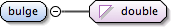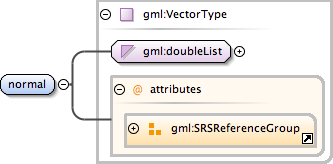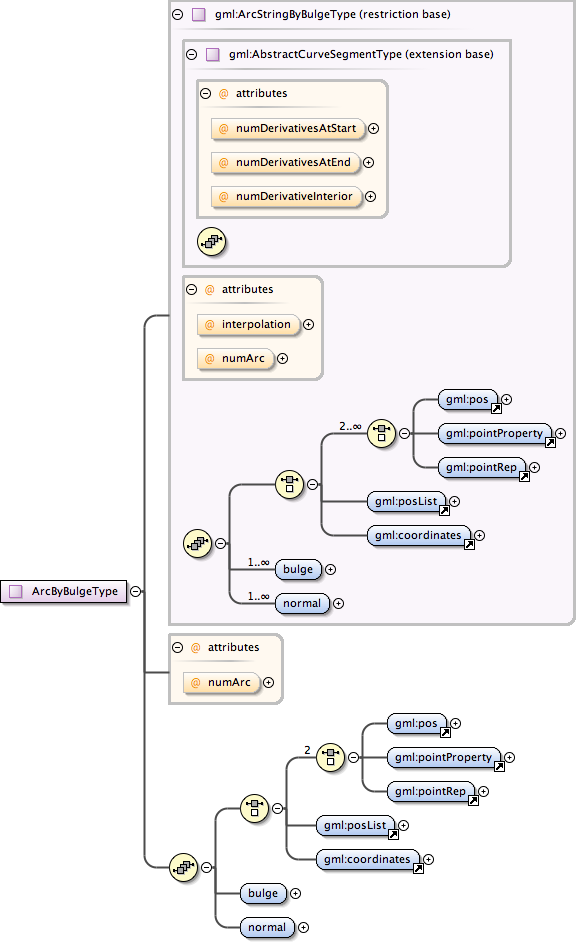### Showing:

 Annotations Attributes Diagrams Properties Source Used by
Element gml:ArcByBulgeType / gml:bulge
Namespace http://www.opengis.net/gml
Annotations
 ```The bulge controls the offset of each arc's midpoint. The "bulge" is the real number multiplier for the normal that determines the offset direction of the midpoint of each arc. The length of the bulge sequence is exactly 1 less than the length of the control point array, since a bulge is needed for each pair of adjacent points in the control point array. The bulge is not given by a distance, since it is simply a multiplier for the normal. The midpoint of the resulting arc is given by: midPoint = ((startPoint + endPoint)/2.0) + bulge*normal```
DiagramType double
Properties
 content: simple
Source
 ``` The bulge controls the offset of each arc's midpoint. The "bulge" is the real number multiplier for the normal that determines the offset direction of the midpoint of each arc. The length of the bulge sequence is exactly 1 less than the length of the control point array, since a bulge is needed for each pair of adjacent points in the control point array. The bulge is not given by a distance, since it is simply a multiplier for the normal. The midpoint of the resulting arc is given by: midPoint = ((startPoint + endPoint)/2.0) + bulge*normal ```
Schema location http://schemas.opengis.net/gml/3.1.1/base/geometryPrimitives.xsd
Element gml:ArcByBulgeType / gml:normal
Namespace http://www.opengis.net/gml
Annotations
 ```The attribute "normal" is a vector normal (perpendicular) to the chord of the arc, the line joining the first and last point of the arc. In a 2D coordinate system, there are only two possible directions for the normal, and it is often given as a signed real, indicating its length, with a positive sign indicating a left turn angle from the chord line, and a negative sign indicating a right turn from the chord. In 3D, the normal determines the plane of the arc, along with the start and endPoint of the arc. The normal is usually a unit vector, but this is not absolutely necessary. If the normal is a zero vector, the geometric object becomes equivalent to the straight line between the two end points. The length of the normal sequence is exactly the same as for the bulge sequence, 1 less than the control point sequence length.```
DiagramType gml:VectorType
Type hierarchy
Properties
 content: complex
Attributes
QName Type Fixed Default Use Annotation
axisLabels gml:NCNameList optional
 ```Ordered list of labels for all the axes of this CRS. The gml:axisAbbrev value should be used for these axis labels, after spaces and forbiddden characters are removed. When the srsName attribute is included, this attribute is optional. When the srsName attribute is omitted, this attribute shall also be omitted.```
srsDimension positiveInteger optional
 ```The "srsDimension" is the length of coordinate sequence (the number of entries in the list). This dimension is specified by the coordinate reference system. When the srsName attribute is omitted, this attribute shall be omitted.```
srsName anyURI optional
 ```In general this reference points to a CRS instance of gml:CoordinateReferenceSystemType (see coordinateReferenceSystems.xsd). For well known references it is not required that the CRS description exists at the location the URI points to. If no srsName attribute is given, the CRS must be specified as part of the larger context this geometry element is part of, e.g. a geometric element like point, curve, etc. It is expected that this attribute will be specified at the direct position level only in rare cases.```
uomLabels gml:NCNameList optional
 ```Ordered list of unit of measure (uom) labels for all the axes of this CRS. The value of the string in the gml:catalogSymbol should be used for this uom labels, after spaces and forbiddden characters are removed. When the axisLabels attribute is included, this attribute shall also be included. When the axisLabels attribute is omitted, this attribute shall also be omitted.```
Source
 ``` The attribute "normal" is a vector normal (perpendicular) to the chord of the arc, the line joining the first and last point of the arc. In a 2D coordinate system, there are only two possible directions for the normal, and it is often given as a signed real, indicating its length, with a positive sign indicating a left turn angle from the chord line, and a negative sign indicating a right turn from the chord. In 3D, the normal determines the plane of the arc, along with the start and endPoint of the arc. The normal is usually a unit vector, but this is not absolutely necessary. If the normal is a zero vector, the geometric object becomes equivalent to the straight line between the two end points. The length of the normal sequence is exactly the same as for the bulge sequence, 1 less than the control point sequence length. ```
Schema location http://schemas.opengis.net/gml/3.1.1/base/geometryPrimitives.xsd
Complex Type gml:ArcByBulgeType
Namespace http://www.opengis.net/gml
Annotations
 `An ArcByBulge is an arc string with only one arc unit, i.e. two control points and one bulge.`
DiagramType restriction of gml:ArcStringByBulgeType
Type hierarchy
Used by
 Element gml:ArcByBulge
Model (gml:pos | gml:pointProperty | gml:pointRep | gml:posList | gml:coordinates) , gml:bulge , gml:normal
Children gml:bulge, gml:coordinates, gml:normal, gml:pointProperty, gml:pointRep, gml:pos, gml:posList
Attributes
QName Type Fixed Default Use Annotation
interpolation gml:CurveInterpolationType circularArc2PointWithBulge optional
 ```The attribute "interpolation" specifies the curve interpolation mechanism used for this segment. This mechanism uses the control points and control parameters to determine the position of this curve segment. For an ArcStringByBulge the interpolation is fixed as "circularArc2PointWithBulge".```
numArc integer 1 optional
 `An arc is an arc string consiting of a single arc, the attribute is fixed to "1".`
numDerivativeInterior integer 0 optional
 ```The attribute "numDerivativesInterior" specifies the type of continuity that is guaranteed interior to the curve. The default value of "0" means simple continuity, which is a mandatory minimum level of continuity. This level is referred to as "C 0 " in mathematical texts. A value of 1 means that the function and its first derivative are continuous at the appropriate end point: "C 1 " continuity. A value of "n" for any integer means the function and its first n derivatives are continuous: "C n " continuity. NOTE: Use of these values is only appropriate when the basic curve definition is an underdetermined system. For example, line string segments cannot support continuity above C 0 , since there is no spare control parameter to adjust the incoming angle at the end points of the segment. Spline functions on the other hand often have extra degrees of freedom on end segments that allow them to adjust the values of the derivatives to support C 1 or higher continuity.```
numDerivativesAtEnd integer 0 optional
 ```The attribute "numDerivativesAtEnd" specifies the type of continuity between this curve segment and its successor. If this is the last curve segment in the curve, one of these values, as appropriate, is ignored. The default value of "0" means simple continuity, which is a mandatory minimum level of continuity. This level is referred to as "C 0 " in mathematical texts. A value of 1 means that the function and its first derivative are continuous at the appropriate end point: "C 1 " continuity. A value of "n" for any integer means the function and its first n derivatives are continuous: "C n " continuity. NOTE: Use of these values is only appropriate when the basic curve definition is an underdetermined system. For example, line string segments cannot support continuity above C 0 , since there is no spare control parameter to adjust the incoming angle at the end points of the segment. Spline functions on the other hand often have extra degrees of freedom on end segments that allow them to adjust the values of the derivatives to support C 1 or higher continuity.```
numDerivativesAtStart integer 0 optional
 ```The attribute "numDerivativesAtStart" specifies the type of continuity between this curve segment and its predecessor. If this is the first curve segment in the curve, one of these values, as appropriate, is ignored. The default value of "0" means simple continuity, which is a mandatory minimum level of continuity. This level is referred to as "C 0 " in mathematical texts. A value of 1 means that the function and its first derivative are continuous at the appropriate end point: "C 1 " continuity. A value of "n" for any integer means the function and its first n derivatives are continuous: "C n " continuity. NOTE: Use of these values is only appropriate when the basic curve definition is an underdetermined system. For example, line string segments cannot support continuity above C 0 , since there is no spare control parameter to adjust the incoming angle at the end points of the segment. Spline functions on the other hand often have extra degrees of freedom on end segments that allow them to adjust the values of the derivatives to support C 1 or higher continuity.```
Source
 ``` An ArcByBulge is an arc string with only one arc unit, i.e. two control points and one bulge. GML supports two different ways to specify the control points of a curve segment. 1. A sequence of "pos" (DirectPositionType) or "pointProperty" (PointPropertyType) elements. "pos" elements are control points that are only part of this curve segment, "pointProperty" elements contain a point that may be referenced from other geometry elements or reference another point defined outside of this curve segment (reuse of existing points). 2. The "posList" element allows for a compact way to specifiy the coordinates of the control points, if all control points are in the same coordinate reference systems and belong to this curve segment only. The number of direct positions in the list must be two. Deprecated with GML version 3.1.0. Use "pointProperty" instead. Included for backwards compatibility with GML 3.0.0. Deprecated with GML version 3.1.0. Use "posList" instead. The bulge controls the offset of each arc's midpoint. The "bulge" is the real number multiplier for the normal that determines the offset direction of the midpoint of each arc. The length of the bulge sequence is exactly 1 less than the length of the control point array, since a bulge is needed for each pair of adjacent points in the control point array. The bulge is not given by a distance, since it is simply a multiplier for the normal. The midpoint of the resulting arc is given by: midPoint = ((startPoint + endPoint)/2.0) + bulge*normal The attribute "normal" is a vector normal (perpendicular) to the chord of the arc, the line joining the first and last point of the arc. In a 2D coordinate system, there are only two possible directions for the normal, and it is often given as a signed real, indicating its length, with a positive sign indicating a left turn angle from the chord line, and a negative sign indicating a right turn from the chord. In 3D, the normal determines the plane of the arc, along with the start and endPoint of the arc. The normal is usually a unit vector, but this is not absolutely necessary. If the normal is a zero vector, the geometric object becomes equivalent to the straight line between the two end points. The length of the normal sequence is exactly the same as for the bulge sequence, 1 less than the control point sequence length. An arc is an arc string consiting of a single arc, the attribute is fixed to "1". ```
Schema location http://schemas.opengis.net/gml/3.1.1/base/geometryPrimitives.xsd
Attribute gml:ArcByBulgeType / @numArc
Namespace No namespace
Annotations
 `An arc is an arc string consiting of a single arc, the attribute is fixed to "1".`
Type integer
Properties
 use: optional fixed: 1
Used by
 Complex Type gml:ArcByBulgeType
Source
 ``` An arc is an arc string consiting of a single arc, the attribute is fixed to "1". ```
Schema location http://schemas.opengis.net/gml/3.1.1/base/geometryPrimitives.xsd